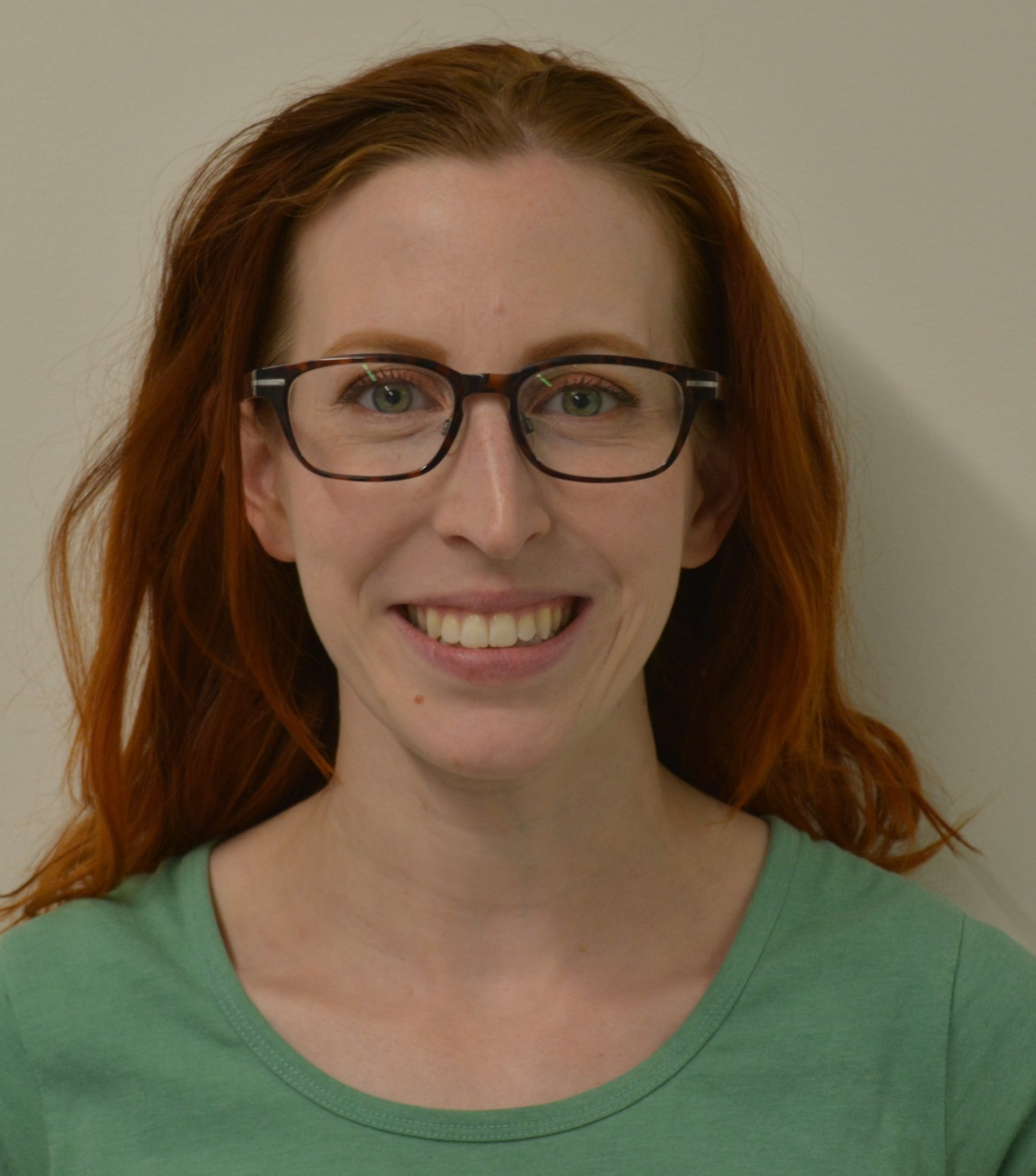# Quantum measurements

By Sarah Plosker, Darian McLaren, and Chris Ramsey
December 2018
Print Version

## What you need to know

Likely, you already know about probability measures. For example, in the word quantum, the probability of choosing the letter q at random is 1/7, the probability of choosing the letter u is 2/7, the probability of choosing the letter a at random is 1/7, and so on, so that all the (positive) probabilities add to 1. A quantum measurement, also called a quantum probability measure, is a set of matrices (a matrix is an array of numbers, organized into rows and columns) that are positive in a certain way, and they add up to the identity matrix, which is a generalization of the number 1. We can take this generalization one step further by imagining infinite-dimensional matrices, with an infinite number of rows and columns.

## Why this research is important

Natural questions (well, natural to a mathematician at least) are: What kind of structure do these objects have? Do they have nice properties? How can I compare them? Can I construct more of these objects by performing mathematical operations on only a few special ones?

Quantum measurements arise naturally in quantum mechanics, and there are underlying statistical principals governing their behaviour. If we can understand them mathematically, we can get closer to understanding the world around us.

## How this research was conducted

This work is at the intersection of several different fields of study, including matrix analysis, operator theory, and quantum information theory. Tools and techniques from these and other areas of mathematics were used to build up our understanding of the mathematical properties of these objects.

## What the researchers found

We were able to generalize some results from classical measure theory to the setting of quantum measurements, and found some entirely new results that do not have a classical analogue (that is, they represent entirely ‘quantum’ results). We also developed a quantum expected value for the infinite-dimensional setting (‘expected value’ is another name for the mean or average; here we are taking the average of infinite-dimensional matrices, which generalizes the usual average of numbers). One subtle issue that becomes an important factor of consideration is that of convergence: in classical probability theory, we have a few small positive numbers (e.g., 1/7, 2/7, 1/7, …) that add up to one. When we generalize this, we can have an infinite number of small positive numbers (or matrices, or other objects) that we are trying to add together. Even though they are very small, there are so many of them that when we add them up, we might get infinity (which we want to avoid).

## How this research can be used

As is often the case in mathematics, the solution to one question raises further questions, and it is always interesting to see how far we can push the results. Some of our results can be used to compare different quantum measures in terms of which quantum measure is ‘bigger’ or ‘better’ than another (in some mathematical sense). Besides being useful information for physicists working in quantum mechanics, these results are of independent interest in pure mathematics; many questions motivated by quantum mechanics represent new lines of inquiry in pure math.

## Acknowledgements

Dr. Plosker’s research is supported by a Natural Sciences and Engineering Research Council (NSERC) Discovery Grant, the Canada Foundation for Innovation, and the Canada Research Chairs Program.### Sarah Plosker, Ph.D.

Ploskers@brandonu.ca

Dr. Sarah Plosker is an Associate Professor Canada Research Chair in Quantum Information Theory in the Department of Mathematics & Computer Science at Brandon University.

### Darian McLaren, Student

Darian McLaren is an undergraduate student at Brandon University doing a joint honours degree in Mathematics and Physics. This research was done during his fourth year honours topics course with Dr. Plosker.

### Chris Ramsey, Ph.D.

Dr. Chris Ramsey was a Postdoctoral Fellow under Dr. Plosker’s supervision in 2017-18, and is now an Assistant Professor in the Department of Mathematics and Statistics at MacEwan University.

## Keywords

• operator-valued measure
• quantum measurement
• quantum probability measure

## Publications Based on the Research

McLaren, D., Plosker, S., & Ramsey, C. (2018). On operator-valued measures. Manuscript submitted for publication.

Plosker, S., & Ramsey, C. (2019). An operator-valued Lyapunov theorem. Journal of Mathematical Analysis and Applications, 469(1), 117–125.

Editor: Christiane Ramsey